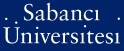# On permutation polynomials over finite fields /

Aksoy, Esen (2006) On permutation polynomials over finite fields /. [Thesis]PDF - Registered users only - Requires a PDF viewer such as GSview, Xpdf or Adobe Acrobat Reader340Kb

## Abstract

A permutation polynomial (PP) over a nite eld Fq is a polynomial in Fq[x] which induces a bijective map from Fq to itself. PPs are of great theoretical interest and are also needed for applications. This thesis starts with some basic facts about PPs. Recent results about one of the most important open problems in this topic: counting PPs of a given degree, are presented. Well known classes of PPs are the linear polynomials, the monomials xk, with gcd(k, q 1) = 1, the linearized polynomials, and the Dickson polynomials. It turns out that nding new classes of PPs is not easy. We also focus on this problem and give a survey of some recent constructions.

Item Type: Thesis Permutation polynomials -- Finite fields -- Binomials -- Monomials -- Dickson polynomials -- symmetric group of degree n. Q Science > QA Mathematics 8350 IC-Cataloging 16 Apr 2008 12:29 27 Dec 2008 13:34

Repository Staff Only: item control page